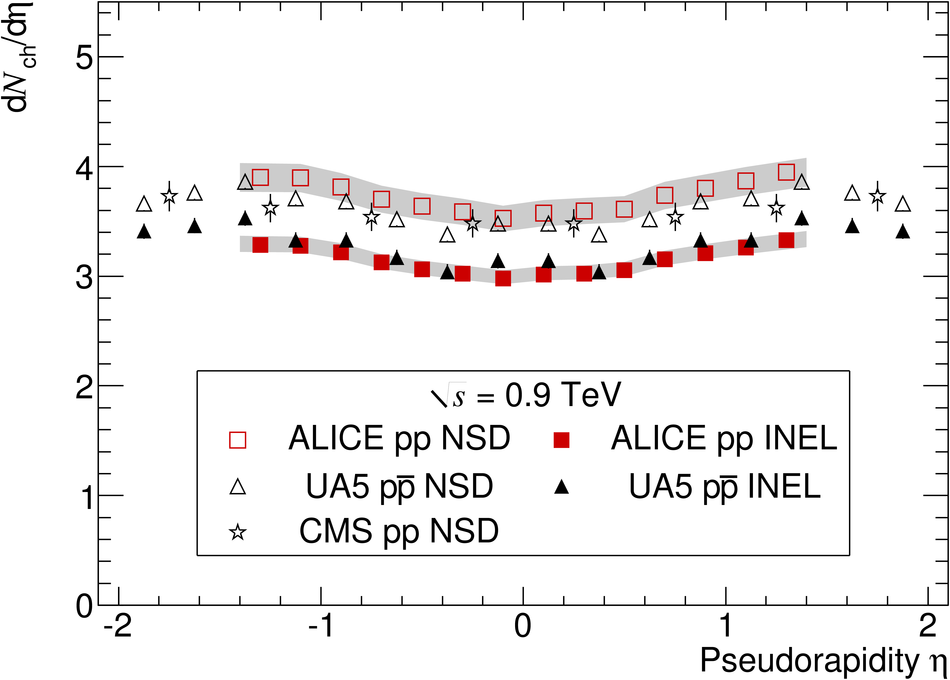# Figure 6

 Top: Measured pseudorapidity dependence of $\dNdEta$ at $\sqrt{s} = 0.9~$TeV for INEL (full symbols) and NSD (open symbols) collisions. The ALICE measurements (squares) are compared to UA5 p$\bar{\mbox{p}}$ data  (triangles) and to CMS pp data at the LHC  (stars). Bottom: Measured pseudorapidity dependence of $\dNdEta$ at $\sqrt{s} = 2.36~$TeV for INEL (full symbols) and NSD (open symbols) collisions. The ALICE measurement (squares) for NSD collisions is compared to CMS NSD data  (stars) and to model predictions, PYTHIA tune D6T  (solid line) and PHOJET  (dashed line). For the ALICE data, systematic uncertainties are shown as shaded areas; statistical uncertainties are invisible (smaller than data marks). For CMS data error bars show the statistical and systematic uncertainties added in quadrature.